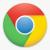## 继续：关于线程的问题 还是有点想不明白

```package net.oschina.tester;

import java.util.*;

public class Test {

public static void main(String[] args) {
final List<Integer> src = new ArrayList<Integer>(){{//源容器
for(int i=1;i<=10;i++)
add(i);
}};
final List<Integer> dest = new ArrayList<Integer>();//目标容器

new Thread(){
int count_once = 2;//每次放2个元素
int pos = 0;
@Override
public void run() {
while(true){
for(int i=0;i<count_once && pos<src.size();i++){
dest.add(src.get(pos++));
}
//间隔时间60毫秒
try {
Thread.sleep(60);
} catch (InterruptedException e) {
break;
}
System.out.printf("pos=%d\n",pos);
if(pos >= src.size())
break;
}
}

}.start();
}

}```

0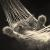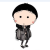00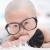`21行  src.get(pos++)  改成 src.remove(pos++) `

0```		new Thread() {
@Override
public void run() {
for(int i=0;i<src.size();i++){
System.out.print(src.get(i));
}
System.out.print('\n');
while (true) {
for(int j=0;j<2;j++){
dest.add(src.get(0));
System.out.println(src.get(0));
src.remove(0);
}
// 间隔时间60毫秒
try {
Thread.sleep(600);
} catch (InterruptedException e) {
break;
}
if (src.size() == 0)
break;
}
}

}.start();```@kxh : 还是有一些小问题的，是在用多线程了。。。这里是用了单线程，还有其他的地方是用了多线程。O(∩_∩)O哈哈~有不会的继续问~\(≧▽≦)/~啦啦啦@黑菜妞妞 : 感觉这东西和线程是没有关系的啊?你要是多线程的话,应该对共享资源src进行加锁的吧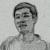@黑菜妞妞 : 栈是先进后出的，这种是模拟队列，先进先出。本来也应该是用队列来做的。

0```多线程测试,虽然多线程时,的确耗时少了,可是当线程过多时,比如1000,感觉有点儿莫名其秒?

public class Test2 implements Runnable {
public long total_times = 0;

final List<Integer> src = new ArrayList<Integer>() {
private static final long serialVersionUID = 6003275546949774361L;
{// 源容器
for (int i = 1; i <= 100000; i++)
add(i);
}
};
final List<Integer> dest = new ArrayList<Integer>();// 目标容器

public void run() {
while (true) {
if (src.size() == 0)
break;
for (int j = 0; j < 2; j++) {
synchronized (src) {
if (src.size() > 0) {
dest.add(src.get(0));
src.remove(0);
} else {
break;
}
}
}
// 间隔时间60毫秒
try {
Thread.sleep(0); // 可以调节此参数试试
} catch (InterruptedException e) {
break;
}
}
}

public static void main(String[] args) throws InterruptedException {
Test2 test = new Test2();

// 线程数,可以调节测试10-100-500?
int thread_count = 10;

for (int i = 0; i < thread_count; i++) {
new Thread(test, "t" + i).start();
}

long start = System.currentTimeMillis();
while (true) {
if (test.src.size() == 0) {
break;
}
}
long end = System.currentTimeMillis();
System.out.println("所有线程累计执行时间:" + (end-start));
}
}```